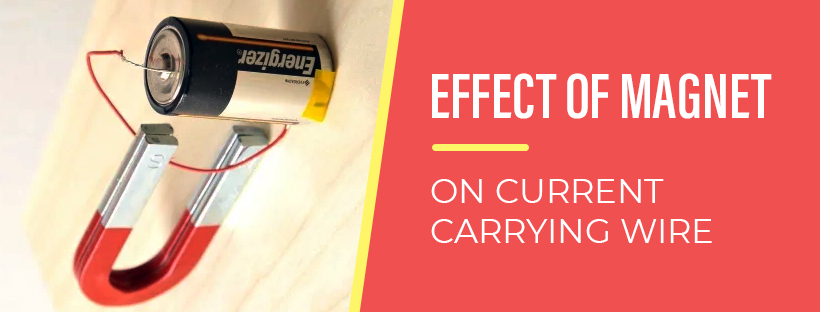Effect of Magnet on Current carrying Wire - Study24x7Default error msg

New to Study24x7 ?

# Effect of Magnet on Current carrying Wire

Updated on 21 January 2022Class12NonMedical
Updated on 21 January 2022Magnetic Force is defined as the force or the influence of a magnet on electric currents. The effect of a magnetic field is seen in permanent magnets like iron which attracts or repels the object. Magnetic field changes with the change in location. The electric currents are created through the magnetic fields with the help of electromagnets and wires.

The magnetic force on a current carrying wire problem is calculated by F=I→l×→B.

A magnetic force acts on a current carrying conductor because the current or the magnetic force is transmitted to the conductor. This is based on the right-hand rule.

There is also a formula for calculating the magnetic field due to current carrying wire. This is calculated by F=Bilsinθ F = Bi l sin θ. In this formula, ‘l’ is the length of the wire, ‘i’ is the current and θ is the angle between the current and the magnetic field. In the case of the magnetic field due to the current carrying conductor, the current is reversed. It in turn encircles the conductor and is perpendicular to it.

Now students often wonder, what is the force of the current carrying conductor in a magnetic field experiment? Well, the force of the current carrying conductor in a magnetic field experiment is calculated by F = IlB sin θ. A conductor experiences a force in a magnetic field experiment. This is determined by Fleming's left-hand rule. The left-hand rule is the way to determine the motion of a conductor when the magnetic field direction and the current direction are known.

## What happens when a current carrying conductor is placed in a magnetic field?

This is a valid question and is often asked by the students. If the current carrying conductor is placed in a magnetic field, then a mechanical and a magnetic force or pressure is exerted which results in the conductor moving a little from its initial position. The force is exerted in the right direction as per Fleming’s left-hand rule as stated above (basically in a reverse direction).Students are often in confusion regarding the effect of the magnetic field produced by a current carrying conductor. Well, the answer to this question will be that the magnetic field is produced by the electric current. The magnetic field is represented as a circular diagram (concentric circles). The direction of the magnetic field can be measured by a compass. In the pictorial representation, it is represented as a straight line intersecting the circles in the middle. It is shown in the diagram below.Now, let’s talk about the force on a current carrying conductor in a magnetic field Class 12th. Students are often confused as to which force is exerted mechanical, magnetic or some other force. The formula has also been stated above to calculate the force.

The formula is F = IlB sin θ. The force exerted is a magnetic force. There is one more way to calculate the force with the help of Fleming’s right-hand rule. The right-hand rule is undertaken to find the direction of the force exerted on a positive moving charge. The formula to calculate this force is F = (qvdB sin θ) (nAl). In this formula, A is the area covered by the wire in the cross-section.

The effect of a magnet on a current carrying wire is one of the important topics in Physics. Students must know the detailed explanation of the magnetism topic. There are various topics covered in this chapter like the magnetic force on a current carrying wire problem and many more. You can take help from reference books or online articles if you have any doubts. Thus, magnetism is one of the important chapters and students must be through with it to attempt this topic easily in the exam.

Related Article:

Difference between Distance and Displacement

Write a comment...Trending ArticlesBy CBSE CLASS 8By Edubabble LLPBy CBSE CLASS 7By CBSE CLASS 10
Related PostsServicesNeed Some Help!Connect